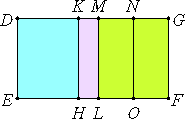# Proposition 65

The square on the side of the sum of two medial areas applied to a rational straight line produces as breadth the sixth binomial.

Let AB be the side of the sum of two medial areas, divided at C, let DE be a rational straight line, and let there be applied to DE the parallelogram DF equal to the square on AB, producing DG as its breadth.

I say that DG is a sixth binomial straight line.

Make the same construction as before.X.41

Since AB is the side of the sum of two medial areas divided at C, therefore AC and CB are straight lines incommensurable in square which make the sum of the squares on them medial, the rectangle contained by them medial, and moreover the sum of the squares on them incommensurable with the rectangle contained by them.

X.22

So that, in accordance with what was before proved, each of the rectangles DL and MF is medial. And they are applied to the rational straight line DE, therefore each of the straight lines DM and MG is rational and incommensurable in length with DE.

Since the sum of the squares on AC and CB is incommensurable with twice the rectangle AC by CB, therefore DL is incommensurable with MF.

Therefore DM is also incommensurable with MG.

X.36

Therefore DM and MG are rational straight lines commensurable in square only. Therefore DG is binomial.

I say next that it is a sixth binomial straight line.

Similarly again we can prove that the rectangle DK by KM equals the square on MN, and that DK is incommensurable in length with KM, and, for the same reason, the square on DM is greater than the square MG by the square on a straight line incommensurable in length with DM.

And neither of the straight lines DM nor MG is commensurable in length with the rational straight line DE set out.

Therefore DG is a sixth binomial straight line.

Therefore, the square on the side of the sum of two medial areas applied to a rational straight line produces as breadth the sixth binomial.

Q.E.D.

## Guide

This proposition is used in X.72.